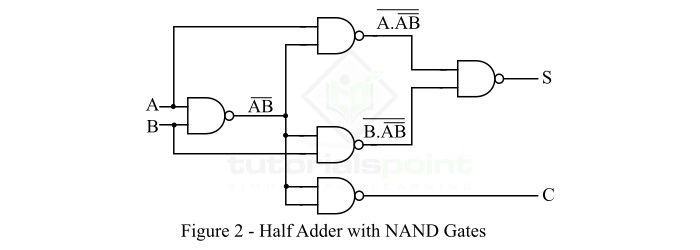# Half Adder with NAND Gates

In digital electronics, there are different types of logic circuits used to perform different kinds of arithmetic operations. One of them is adder. Adder (or Binary Adder) is a combinational logic circuit that performs the addition of two or more binary numbers and gives an output sum. There are two types of adders present namely, half adder and full adder.

Since, adder are logic circuits, thus they are implemented using different types of digital logic gates such as OR gate, AND gate, NOT gate, NAND gates, etc. In this article, we will discuss the Half Adder Realization using NAND Gates. But before that let’s have a look into the basics of half adder.

A combinational logic circuit which is designed to add two binary digits is called as a half adder. The half adder provides the output along with a carry value (if any). The half adder circuit is designed by connecting an EX-OR gate and one AND gate. It has two input terminals and two output terminals for sum and carry. The block diagram and circuit diagram of a half adder are shown in Figure-1.In the block diagram of the half adder, A and B are the input variables, S is the output sum bit, and C is the output carry bit.

## Truth Table of Half Adder

The following is the truth table of the half-adder −

Inputs Outputs
A B S (Sum) C (Carry)
0 0 0 0
0 1 1 0
1 0 1 0
1 1 0 1

From the truth table of half adder, we can find the output equations for Sum (S) and Carry (C) bits. These output equations are given below −

The sum (S) of the half-adder is,

$$\mathrm{Sum,\, S=AB'+A'B }$$

The carry (C) of the half-adder is,

$$\mathrm{Carry,\, C=A\cdot B }$$

## Half Adder with NAND Gates

We can implement the half adder circuit using NAND gates. The NAND gate is basically a universal gate, i.e. it can be used for designing any digital circuit. The realization of half adder with NAND gate is shown in Figure-2.From the circuit of half adder with NAND gate, it is clear that the minimum of 5 NAND gates are required to design a half adder circuit.

Here, we can see that the first NAND gate takes the input bits A and B. The output of the first NAND gate is again given as the input to 3 NAND gates along with the original inputs. Out of the three NAND gates, 2 NAND gates produce the outputs that are again given as the input to the NAND gate which is connected at the end of the circuit. This NAND gate at the end of the circuit gives the sum bit (S). Out of the three NAND gates at the second stage, the third NAND gate generates the carry bit (C).

The operation of the circuit of half adder with NAND gates can be understood more clearly with the help of following equations −

$$\mathrm{Sum,\, S=((A \cdot (AB)')' \cdot (B \cdot (AB)')')'}$$

$$\mathrm{\Rightarrow Sum,\, S=((A \cdot (AB)')')' + ((B \cdot (AB)')')'}$$

$$\mathrm{\Rightarrow Sum,\, S=A \cdot (AB)' + B \cdot (AB)'}$$

$$\mathrm{\Rightarrow Sum,\, S=A \cdot (A'+B') + B \cdot (A'+B')}$$

$$\mathrm{\Rightarrow Sum,\, S=AA'+AB'+A'B+BB'}$$

$$\mathrm{\therefore Sum,\, S=AB'+A'B=A\oplus B}$$

Similarly, the carry bit (C) is given by,

$$\mathrm{Carry,\, C=((AB)')'=AB}$$

Hence, in this way we can also realize the half adder in NAND logic.